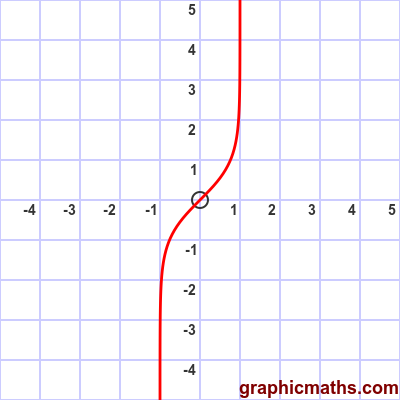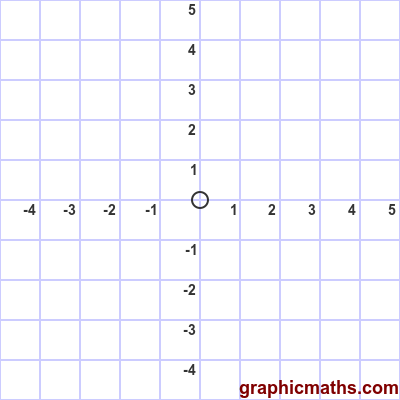# artanh function

Martin McBride, 2020-02-03
Tags artanh
Categories hyperbolic functions

The artanh function is a hyperbolic function. It is the inverse of the tanh, and is also known as the inverse hyperbolic tangent function.

## Equation and graph

The artanh function is defined as the inverse of tanh, ie if:

$$x = \tanh y$$

then:

$$y = \operatorname{artanh} x$$

There is a also a formula for finding artanh directly:

$$\operatorname{artanh} x =\frac12\ln\left(\frac{1+x}{1-x}\right)$$

Here is a graph of the function:The function is only valid for -1 < x < 1.

## artanh as inverse of tanh

This animation illustrates the relationship between the tanh function and the artanh function:The first, blue, curve is the tanh function.

The grey dashed line is the line $y=x$.

The second, red, curve is the artanh function. As with any inverse function, it is identical to the original function reflected in the line $y=x$.